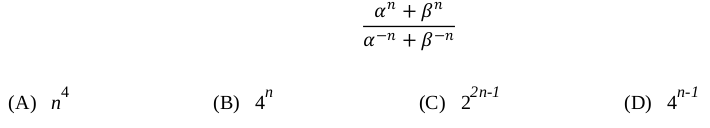Related Articles

# GATE | GATE-CS-2016 (Set 2) | Question 5

• Last Updated : 28 Jun, 2021

In a quadratic function, the value of the product of the roots (α, β) is 4. Find the value of(A) A
(B) B
(C) C
(D) D

Explanation:
(α^n + β^n)/ (1/α^n +1/β^n)

(α^n + β^n)/ (β^n + α^n )*(α^n * β^n)

(α^n * β^n)

αβ^n

4^n

This explanation has been contributed by Dharmesh Singh.

Quiz of this Question

Attention reader! Don’t stop learning now.  Practice GATE exam well before the actual exam with the subject-wise and overall quizzes available in GATE Test Series Course.

Learn all GATE CS concepts with Free Live Classes on our youtube channel.

My Personal Notes arrow_drop_up